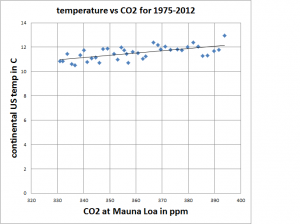Jul 07

## Global Temperature-meaning of Slope and y-intercept

This is a problem I work through in my pre-calculus class when we are discussing the meaning of the slope and y-intercept.  It also gives me the opportunity to discuss the perils of extrapolating far outside of the data set.

Consider the following data and the linear model that fits it.The equation for the linear model is y=0.019x+4.76.  Answer the following questions in complete sentences with units.

1. What are the units for the slope?
2. What does the slope mean in this particular situation?
3. What are the units for the y-intercept?
4. What does the y-intercept mean in this particular situation?

(data from http://www.esrl.noaa.gov/gmd/dv/data/index.php?site=mlo&parameter_name=Carbon%2BDioxide&frequency=Monthly%2BAverages and http://www.ncdc.noaa.gov/cag/ )

XHTML: You can use these tags: <a href="" title=""> <abbr title=""> <acronym title=""> <b> <blockquote cite=""> <cite> <code> <del datetime=""> <em> <i> <q cite=""> <s> <strike> <strong>